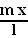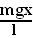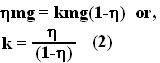## Saturday, February 16, 2008

### Irodov Problem 1.124Suppose that some instant x is the length of the chain remaining on the table. The mass of this part of the chain (assuming the chain is homogenous) is given byand hence the force of gravity acting on this piece is.

In the vertical direction there are two forces acting on the chain, i) the force of gravity and ii) the normal reaction from the surface N. There is no acceleration for the chain in the vertical direction and so we have,Since the chain is slipping, the maximum force of friction that can be offered by the rough surface has been attained and is equal to Nk, here k is the coefficient of friction.

The problem states that only when initiallylength of the chain hangs, the chain will start slipping. The force of gravity that is responsible for pulling the chain downwards at this initial time is given by. At this time. So from (1) then the force of friction opposing the motion of the chain will be. This means that in order forto be the minimum hanging length to slip we have,As the chain fall lesser and lesser length of the chain remains on the table. This means that the normal reaction offered by the table surface keeps decreasing as the chain falls. Since the force o friction is given by Nk, even the force of friction keeps decreasing. When x length of the chain remains on the table, the force of friction offered by the surface of the table is given by,The work done by friction is thus given by,The negative sign comes because at all points when the chain slips, the force of friction acts in the direction opposed to the motion of the chain.

#### 1 comment:Anonymous said...

I just stuck at that point that the friction force will decrease as the chain slips!! wonderful, in fact sometime I think You are too descriptive, anyways great work man!